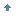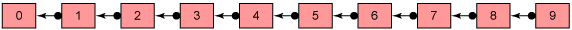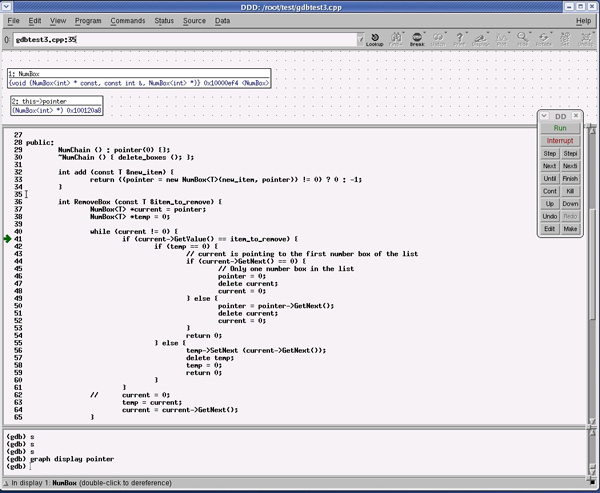# Valgrind使用回页首

Valgrind

Valgrind 已经在 Linux 应用程序开发社区中广泛用来调试应用程序。它尤其擅长发现内存管理的问题。它可以检查程序运行时的内存泄漏问题。这个工具目前正由 Julian Seward 进行开发，并由 Paul Mackerras 移植到了 Power 架构上。

 ```# make # make check # make install ```

Valgrind 的错误报告

Valgrind 的输出格式如下：

 ``` # valgrind du –x –s . . ==29404== Address 0x1189AD84 is 0 bytes after a block of size 12 alloc'd ==29404== at 0xFFB9964: malloc (vg_replace_malloc.c:130) ==29404== by 0xFEE1AD0: strdup (in /lib/tls/libc.so.6) ==29404== by 0xFE94D30: setlocale (in /lib/tls/libc.so.6) ==29404== by 0x10001414: main (in /usr/bin/du) ```

`==29404==` 是进程的 ID。消息 `Address 0x1189AD84 is 0 bytes after a block of size 12 alloc'd` 说明在这个 12 字节的数组后面没有存储空间了。第二行以及后续几行说明内存是在 130 行（vg_replace_malloc.c）的 `strdup()` 程序中进行分配的。`strdup()` 是在 libc.so.6 库的 `setlocale()` 中调用的；`main()` 调用了 `setlocale()`

• 未经初始化的变量
• malloc 函数所分配的数据，在写入值之前使用了

 ``` 2 { 3 int i; 4 5 if (i == 0) 6 i=1; 7 return 0; 8 } ```

 ``` # gcc –g –o test1 test1.c # valgrind ./test1 . . ==31363== ==31363== Conditional jump or move depends on uninitialised value(s) ==31363== at 0x1000041C: main (test1.c:5) ==31363== ==31363== ERROR SUMMARY: 1 errors from 1 contexts (suppressed: 7 from 1) ==31363== malloc/free: in use at exit: 0 bytes in 0 blocks. ==31363== malloc/free: 0 allocs, 0 frees, 0 bytes allocated. ==31363== For counts of detected errors, rerun with: -v ==31363== No malloc'd blocks -- no leaks are possible. ```

Valgrind 的输出说明，有一个条件分支依赖于文件 test1.c 中第 5 行中的一个未初始化的变量。

 ``` 1 int main(void) 2 { 3 char *p1; 4 char *p2; 5 6 p1 = (char *) malloc(512); 7 p2 = (char *) malloc(512); 8 9 p1=p2; 10 11 free(p1); 12 free(p2); 13 } ```

 ``` # gcc –g –o test2 test2.c # valgrind ./test2 . . ==31468== Invalid free() / delete / delete[] ==31468== at 0xFFB9FF0: free (vg_replace_malloc.c:152) ==31468== by 0x100004B0: main (test2.c:12) ==31468== Address 0x11899258 is 0 bytes inside a block of size 512 free'd ==31468== at 0xFFB9FF0: free (vg_replace_malloc.c:152) ==31468== by 0x100004A4: main (test2.c:11) ==31468== ==31468== ERROR SUMMARY: 1 errors from 1 contexts (suppressed: 7 from 1) ==31468== malloc/free: in use at exit: 512 bytes in 1 blocks. ==31468== malloc/free: 2 allocs, 2 frees, 1024 bytes allocated. ==31468== For counts of detected errors, rerun with: -v ==31468== searching for pointers to 1 not-freed blocks. ==31468== checked 167936 bytes. ==31468== ==31468== LEAK SUMMARY: ==31468== definitely lost: 512 bytes in 1 blocks. ==31468== possibly lost: 0 bytes in 0 blocks. ==31468== still reachable: 0 bytes in 0 blocks. ==31468== suppressed: 0 bytes in 0 blocks. ==31468== Use --leak-check=full to see details of leaked memory. ```

 ``` 1 int main() { 2 int i, *iw, *ir; 3 4 iw = (int *)malloc(10*sizeof(int)); 5 ir = (int *)malloc(10*sizeof(int)); 6 7 8 for (i=0; i<11; i++) 9 iw[i] = i; 10 11 for (i=0; i<11; i++) 12 ir[i] = iw[i]; 13 14 free(iw); 15 free(ir); 16 } ```

 ``` # gcc –g –o test3 test3.c # valgrind ./test3 . . ==31522== Invalid write of size 4 ==31522== at 0x100004C0: main (test3.c:9) ==31522== Address 0x11899050 is 0 bytes after a block of size 40 alloc'd ==31522== at 0xFFB9964: malloc (vg_replace_malloc.c:130) ==31522== by 0x10000474: main (test10.c:4) ==31522== ==31522== Invalid read of size 4 ==31522== at 0x1000050C: main (test3.c:12) ==31522== Address 0x11899050 is 0 bytes after a block of size 40 alloc'd ==31522== at 0xFFB9964: malloc (vg_replace_malloc.c:130) ==31522== by 0x10000474: main (test10.c:4) ==31522== ==31522== ERROR SUMMARY: 2 errors from 2 contexts (suppressed: 7 from 1) ==31522== malloc/free: in use at exit: 0 bytes in 0 blocks. ==31522== malloc/free: 2 allocs, 2 frees, 84 bytes allocated. ==31522== For counts of detected errors, rerun with: -v ==31522== No malloc'd blocks -- no leaks are possible. ```

Valgrind 也可以帮助判断内存误用的问题，例如：

• 读/写已经释放的内存
• C++ 环境中错误地使用 malloc/new 与 free/delete 的配对

• memcheck 和 addrcheck 工具都可以很好地工作。然而，其他工具还没有进行大量的测试。另外，Helgrind （一个数据竞争的检测程序）在 POWER 上尚不能使用。
• 所有的 32 位 PowerPC? 用户模式的指令都可以支持，除了两条非常少用的指令：lswx 和 stswx。具体来说，所有的浮点和 Altivec（VMX）指令都可以支持。
• Valgrind 可以在 32 位或 64 位 PowerPC/Linux 内核上工作，但是只能用于 32 位的可执行程序。回页首

GNU 项目调试器

GDB（GNU 项目调试器）可以让您了解程序在执行时“内部” 究竟在干些什么，以及在程序发生崩溃的瞬间正在做什么。

GDB 做以下 4 件主要的事情来帮助您捕获程序中的 bug：

• 在程序启动之前指定一些可以影响程序行为的变量或条件
• 在某个指定的地方或条件下暂停程序
• 在程序停止时检查已经发生了什么
• 在程序执行过程中修改程序中的变量或条件，这样就可以体验修复一个 bug 的成果，并继续了解其他 bug

gdb 中有很多命令。使用 `help` 命令可以列出所有的命令，以及关于如何使用这些命令的介绍。下表给出了最常用的 GDB 命令。

`help` 显示命令类别 `help` - 显示命令类别
`help breakpoints `- 显示属于 breakpoints 类别的命令
`help break` - 显示 break 命令的解释
`run` 启动所调试的程序 ?
`kill` 终止正在调试的程序的执行 通常这会在要执行的代码行已经超过了您想要调试的代码时使用。执行`kill` 会重置断点，并从头再次运行这个程序

`cont` 所调试的程序运行到一个断点、异常或单步之后，继续执行 ?
`info break` 显示当前的断点或观察点 ?
`break` 在指定的行或函数处设置断点 `break 93 if i=8` - 当变量 i 等于 8 时，在第 93 行停止程序执行
`Step` 单步执行程序，直到它到达一个不同的源代码行。您可以使用 `s` 来代表 step 命令 ?
`Next` 与 step 命令类似，只是它不会“单步跟踪到”子例程中 ?
`print` 打印一个变量或表达式的值 `print pointer` - 打印变量指针的内容
`print *pointer` - 打印指针所指向的数据结构的内容
`delete` 删除某些断点或自动显示表达式 `delete 1` - 删除断点 1。断点可以通过 `info break` 来显示
`watch` 为一个表达式设置一个观察点。当表达式的值发生变化时，这个观察点就会暂停程序的执行 ?
`where` 打印所有堆栈帧的栈信息 `where` - 不使用参数，输出当前线程的堆栈信息
`where all` - 输出当前线程组中所有线程的堆栈信息
`where threadindex `- 输出指定线程的堆栈信息
`attach` 开始查看一个已经运行的进程 attach <process_id> - 附加到进程 process_id 上。process_id 可以使用 ps 命令找到
`info thread` 显示当前正在运行的线程 ?
`thread apply threadno command` 对一个线程运行 gdb 命令 `thread apply 3 where` - 对线程 3 运行 `where` 命令
`Thread threadno` 选择一个线程作为当前线程 ?

 `# gdb programname corefilename `

 `# gdb -c core programname `

gdb 会显示是哪行代码导致这个程序产生了核心转储。``` # g++ -g -o gdbtest1 gdbtest1.cpp # ./gdbtest1 Number Box "0" created Number Box "1" created Number Box "2" created Number Box "3" created Number Box "4" created Number Box "5" created Number Box "6" created Number Box "7" created Number Box "8" created Number Box "9" created list created Number Box "9" deleted Segmentation fault ```

 ``` # gdb ./gdbtest1 GNU gdb 6.2.1 Copyright 2004 Free Software Foundation, Inc. GDB is free software, covered by the GNU General Public License, and you are welcome to change it and/or distribute copies of it under certain conditions. Type "show copying" to see the conditions. There is absolutely no warranty for GDB. Type "show warranty" for details. This GDB was configured as "ppc-suse-linux"...Using host libthread_db library "/lib/tls/libthread_db.so.1". (gdb) ```

 ``` (gdb) run Starting program: /root/test/gdbtest1 Number Box "0" created Number Box "1" created Number Box "2" created Number Box "3" created Number Box "4" created Number Box "5" created Number Box "6" created Number Box "7" created Number Box "8" created Number Box "9" created list created Number Box "9" deleted Program received signal SIGSEGV, Segmentation fault. 0x10000f74 in NumBox::GetNext (this=0x0) at gdbtest1.cpp:14 14 NumBox*GetNext() const { return Next; } (gdb) where #0 0x10000f74 in NumBox::GetNext (this=0x0) at gdbtest1.cpp:14 #1 0x10000d10 in NumChain::RemoveBox (this=0x10012008, item_to_remove=@0xffffe200) at gdbtest1.cpp:63 #2 0x10000978 in main (argc=1, argv=0xffffe554) at gdbtest1.cpp:94 (gdb) ```

 ``` 54 } else { 55 temp->SetNext (current->GetNext()); 56 delete temp; 57 temp = 0; 58 return 0; 59 } 60 } 61 current = 0; 62 temp = current; 63 current = current->GetNext(); 64 } 65 66 return -1; ```

 ``` # g++ -g -o gdbtest2 gdbtest2.cpp # ./gdbtest2 Number Box "0" created Number Box "1" created Number Box "2" created Number Box "3" created Number Box "4" created Number Box "5" created Number Box "6" created Number Box "7" created Number Box "8" created Number Box "9" created list created Number Box "9" deleted Number Box "0" deleted ```

 ``` # gdb ./gdbtest2 GNU gdb 6.2.1 Copyright 2004 Free Software Foundation, Inc. GDB is free software, covered by the GNU General Public License, and you are welcome to change it and/or distribute copies of it under certain conditions. Type "show copying" to see the conditions. There is absolutely no warranty for GDB. Type "show warranty" for details. This GDB was configured as "ppc-suse-linux"...Using host libthread_db library "/lib/tls/libthread_db.so.1". (gdb) break 94 if i==8 Breakpoint 1 at 0x10000968: file gdbtest2.cpp, line 94. (gdb) run Starting program: /root/test/gdbtest2 Number Box "0" created Number Box "1" created Number Box "2" created Number Box "3" created Number Box "4" created Number Box "5" created Number Box "6" created Number Box "7" created Number Box "8" created Number Box "9" created list created Number Box "9" deleted Breakpoint 1, main (argc=1, argv=0xffffe554) at gdbtest2.cpp:94 94 list ->RemoveBox(i); ```

 ``` (gdb) s 38 NumBox *temp = 0; (gdb) s 40 while (current != 0) { (gdb) print pointer \$1 = (NumBox *) 0x100120a8 (gdb) print *pointer \$2 = {Num = 0, Next = 0x0} (gdb) ```

 ``` (gdb) kill Kill the program being debugged? (y or n) y (gdb) info break Num Type Disp Enb Address What 1 breakpoint keep y 0x10000968 in main at gdbtest2.cpp:94 stop only if i == 8 breakpoint already hit 1 time (gdb) delete 1 (gdb) break 94 if i==9 Breakpoint 2 at 0x10000968: file gdbtest2.cpp, line 94. (gdb) run Starting program: /root/test/gdbtest2 Number Box "0" created Number Box "1" created Number Box "2" created Number Box "3" created Number Box "4" created Number Box "5" created Number Box "6" created Number Box "7" created Number Box "8" created Number Box "9" created list created Breakpoint 2, main (argc=1, argv=0xffffe554) at gdbtest2.cpp:94 94 list ->RemoveBox(i); (gdb) ```

 ``` Breakpoint 2, main (argc=1, argv=0xffffe554) at gdbtest2.cpp:94 94 list ->RemoveBox(i); (gdb) s NumChain::RemoveBox (this=0x10012008, item_to_remove=@0xffffe200) at gdbtest2.cpp:37 37 NumBox *current = pointer; (gdb) display *pointer 1: *this->pointer = {Num = 9, Next = 0x10012098} (gdb) s 38 NumBox *temp = 0; 1: *this->pointer = {Num = 9, Next = 0x10012098} (gdb) s 40 while (current != 0) { 1: *this->pointer = {Num = 9, Next = 0x10012098} (gdb) s 41 if (current->GetValue() == item_to_remove) { 1: *this->pointer = {Num = 9, Next = 0x10012098} (gdb) s NumBox::GetValue (this=0x100120a8) at gdbtest2.cpp:16 16 const T& GetValue () const { return Num; } (gdb) s NumChain::RemoveBox (this=0x10012008, item_to_remove=@0xffffe200) at gdbtest2.cpp:42 42 if (temp == 0) { 1: *this->pointer = {Num = 9, Next = 0x10012098} (gdb) s 44 if (current->GetNext() == 0) { 1: *this->pointer = {Num = 9, Next = 0x10012098} (gdb) s NumBox::GetNext (this=0x100120a8) at gdbtest2.cpp:14 14 NumBox*GetNext() const { return Next; } (gdb) s NumChain::RemoveBox (this=0x10012008, item_to_remove=@0xffffe200) at gdbtest2.cpp:50 50 delete current; 1: *this->pointer = {Num = 9, Next = 0x10012098} (gdb) s ~NumBox (this=0x100120a8) at gdbtest2.cpp:10 10 std::cout << "Number Box " <<"/"" << GetValue() <<"/"" <<" deleted" << std::endl; (gdb) s NumBox::GetValue (this=0x100120a8) at gdbtest2.cpp:16 16 const T& GetValue () const { return Num; } (gdb) s Number Box "9" deleted ~NumBox (this=0x100120a8) at gdbtest2.cpp:11 11 Next = 0; (gdb) s NumChain::RemoveBox (this=0x10012008, item_to_remove=@0xffffe200) at gdbtest2.cpp:51 51 current = 0; 1: *this->pointer = {Num = 0, Next = 0x0} (gdb) s 53 return 0; 1: *this->pointer = {Num = 0, Next = 0x0} (gdb) s 0x10000d1c 66 return -1; 1: *this->pointer = {Num = 0, Next = 0x0} ```

 ``` 49 } else { 50 pointer = pointer->GetNext(); 51 delete current; 52 current = 0; 53 } 54 return 0; ```

 ``` # g++ -g -o gdbtest3 gdbtest3.cpp # ./gdbtest3 Number Box "0" created Number Box "1" created Number Box "2" created Number Box "3" created Number Box "4" created Number Box "5" created Number Box "6" created Number Box "7" created Number Box "8" created Number Box "9" created list created Number Box "9" deleted Number Box "8" deleted Number Box "7" deleted Number Box "6" deleted Number Box "5" deleted Number Box "4" deleted Number Box "3" deleted Number Box "2" deleted Number Box "1" deleted Number Box "0" deleted ```

 ``` #include #include "pthread.h> pthread_mutex_t AccountA_mutex; pthread_mutex_t AccountB_mutex; struct BankAccount { char account_name; int balance; }; struct BankAccount accountA = {"A", 10000 }; struct BankAccount accountB = {"B", 20000 }; void * transferAB (void* amount_ptr) { int amount = *((int*)amount_ptr); pthread_mutex_lock(&AccountA_mutex); if (accountA.balance < amount) { printf("There is not enough memory in Account A!/n"); pthread_mutex_unlock(&AccountA_mutex); pthread_exit((void *)1); } accountA.balance -=amount; sleep(1); pthread_mutex_lock(&AccountB_mutex); accountB.balance +=amount; pthread_mutex_unlock(&AccountA_mutex); pthread_mutex_unlock(&AccountB_mutex); } void * transferBA (void* amount_ptr) { int amount = *((int*)amount_ptr); pthread_mutex_lock(&AccountB_mutex); if (accountB.balance < amount) { printf("There is not enough memory in Account B!/n"); pthread_mutex_unlock(&AccountB_mutex); pthread_exit((void *)1); } accountB.balance -=amount; sleep(1); pthread_mutex_lock(&AccountA_mutex); accountA.balance +=amount; pthread_mutex_unlock(&AccountB_mutex); pthread_mutex_unlock(&AccountA_mutex); } int main(int argc, char* argv[]) { int threadid; pthread_t pthread; int transfer_amount = {100, 200, 300, 400}; int final_balanceA, final_balanceB; final_balanceA=accountA.balance-transfer_amount- transfer_amount+transfer_amount+transfer_amount; final_balanceB=accountB.balance+transfer_amount +transfer_amount-transfer_amount-transfer_amount; if (threadid = pthread_create(&pthread, NULL, transferAB, (void*)&transfer_amount) " 0) { perror("Thread #0 creation failed."); exit (1); } if (threadid = pthread_create(&pthread, NULL, transferAB, (void*)&transfer_amount) " 0) { perror("Thread #1 creation failed."); exit (1); } if (threadid = pthread_create(&pthread, NULL, transferBA, (void*)&transfer_amount) < 0) { perror("Thread #2 creation failed."); exit (1); } if (threadid = pthread_create(&pthread, NULL, transferBA, (void*)&transfer_amount) < 0) { perror("Thread #3 creation failed."); exit (1); } printf("Transitions are in progress.."); while ((accountA.balance != final_balanceA) && (accountB.balance != final_balanceB)) { printf(".."); } printf("/nAll the money is transferred !!/n"); } ```

 ```# gcc -g -o gdbtest2 gdbtest2.c -L/lib/tls -lpthread ```

 ``` # ps -ef |grep gdbtest2 root 9510 8065 1 06:30 pts/1 00:00:00 ./gdbtest2 root 9516 9400 0 06:30 pts/4 00:00:00 grep gdbtest2 # gdb -pid 9510 GNU gdb 6.2.1 Copyright 2004 Free Software Foundation, Inc. GDB is free software, covered by the GNU General Public License, and you are welcome to change it and/or distribute copies of it under certain conditions. Type "show copying" to see the conditions. There is absolutely no warranty for GDB. Type "show warranty" for details. This GDB was configured as "ppc-suse-linux". Attaching to process 9510 Reading symbols from /root/test/gdbtest2...done. Using host libthread_db library "/lib/tls/libthread_db.so.1". Reading symbols from /lib/tls/libpthread.so.0...done. [Thread debugging using libthread_db enabled] [New Thread 1073991712 (LWP 9510)] [New Thread 1090771744 (LWP 9514)] [New Thread 1086577440 (LWP 9513)] [New Thread 1082383136 (LWP 9512)] [New Thread 1078188832 (LWP 9511)] Loaded symbols for /lib/tls/libpthread.so.0 Reading symbols from /lib/tls/libc.so.6...done. Loaded symbols for /lib/tls/libc.so.6 Reading symbols from /lib/ld.so.1...done. Loaded symbols for /lib/ld.so.1 0x0ff4ac40 in __write_nocancel () from /lib/tls/libc.so.6 (gdb) info thread 5 Thread 1078188832 (LWP 9511) 0x0ffe94ec in __lll_lock_wait () from /lib/tls/libpthread.so.0 4 Thread 1082383136 (LWP 9512) 0x0ffe94ec in __lll_lock_wait () from /lib/tls/libpthread.so.0 3 Thread 1086577440 (LWP 9513) 0x0ffe94ec in __lll_lock_wait () from /lib/tls/libpthread.so.0 2 Thread 1090771744 (LWP 9514) 0x0ffe94ec in __lll_lock_wait () from /lib/tls/libpthread.so.0 1 Thread 1073991712 (LWP 9510) 0x0ff4ac40 in __write_nocancel () from /lib/tls/libc.so.6 (gdb) ```

 ``` (gdb) thread apply 1 where Thread 1 (Thread 1073991712 (LWP 9510)): #0 0x0ff4ac40 in __write_nocancel () from /lib/tls/libc.so.6 #1 0x0ff4ac28 in __write_nocancel () from /lib/tls/libc.so.6 Previous frame identical to this frame (corrupt stack?) #0 0x0ff4ac40 in __write_nocancel () from /lib/tls/libc.so.6 (gdb) thread apply 2 where Thread 2 (Thread 1090771744 (LWP 9514)): #0 0x0ffe94ec in __lll_lock_wait () from /lib/tls/libpthread.so.0 #1 0x0ffe466c in pthread_mutex_lock () from /lib/tls/libpthread.so.0 #2 0x0ffe466c in pthread_mutex_lock () from /lib/tls/libpthread.so.0 #3 0x0ffe466c in pthread_mutex_lock () from /lib/tls/libpthread.so.0 #4 0x0ffe466c in pthread_mutex_lock () from /lib/tls/libpthread.so.0 Previous frame inner to this frame (corrupt stack?) #0 0x0ff4ac40 in __write_nocancel () from /lib/tls/libc.so.6 (gdb) thread apply 3 where Thread 3 (Thread 1086577440 (LWP 9513)): #0 0x0ffe94ec in __lll_lock_wait () from /lib/tls/libpthread.so.0 #1 0x0ffe466c in pthread_mutex_lock () from /lib/tls/libpthread.so.0 #2 0x0ffe466c in pthread_mutex_lock () from /lib/tls/libpthread.so.0 #3 0x0ffe466c in pthread_mutex_lock () from /lib/tls/libpthread.so.0 #4 0x0ffe466c in pthread_mutex_lock () from /lib/tls/libpthread.so.0 Previous frame inner to this frame (corrupt stack?) #0 0x0ff4ac40 in __write_nocancel () from /lib/tls/libc.so.6 (gdb) thread apply 4 where Thread 4 (Thread 1082383136 (LWP 9512)): #0 0x0ffe94ec in __lll_lock_wait () from /lib/tls/libpthread.so.0 #1 0x0ffe466c in pthread_mutex_lock () from /lib/tls/libpthread.so.0 #2 0x0ffe466c in pthread_mutex_lock () from /lib/tls/libpthread.so.0 #3 0x0ffe466c in pthread_mutex_lock () from /lib/tls/libpthread.so.0 #4 0x0ffe466c in pthread_mutex_lock () from /lib/tls/libpthread.so.0 Previous frame inner to this frame (corrupt stack?) #0 0x0ff4ac40 in __write_nocancel () from /lib/tls/libc.so.6 (gdb) thread apply 5 where Thread 5 (Thread 1078188832 (LWP 9511)): #0 0x0ffe94ec in __lll_lock_wait () from /lib/tls/libpthread.so.0 #1 0x0ffe466c in pthread_mutex_lock () from /lib/tls/libpthread.so.0 #2 0x0ffe466c in pthread_mutex_lock () from /lib/tls/libpthread.so.0 #3 0x0ffe466c in pthread_mutex_lock () from /lib/tls/libpthread.so.0 #4 0x0ffe466c in pthread_mutex_lock () from /lib/tls/libpthread.so.0 Previous frame inner to this frame (corrupt stack?) #0 0x0ff4ac40 in __write_nocancel () from /lib/tls/libc.so.6 ```

 ``` (gdb) print AccountA_mutex \$1 = {__m_reserved = 2, __m_count = 0, __m_owner = 0x2527, __m_kind = 0, __m_lock = {__status = 1, __spinlock = 0}} (gdb) print 0x2527 \$2 = 9511 (gdb) print AccountB_mutex \$3 = {__m_reserved = 2, __m_count = 0, __m_owner = 0x2529, __m_kind = 0, __m_lock = {__status = 1, __spinlock = 0}} (gdb) print 0x2529 \$4 = 9513 (gdb) ```

 ``` . . void * transferAB (void* amount_ptr) { int amount = *((int*)amount_ptr); pthread_mutex_lock(&AccountA_mutex); pthread_mutex_lock(&AccountB_mutex); if (accountA.balance < amount) { printf("There is not enough memory in Account A!/n"); pthread_mutex_unlock(&AccountA_mutex); pthread_exit((void *)1); } accountA.balance -=amount; sleep(1); accountB.balance +=amount; pthread_mutex_unlock(&AccountA_mutex); pthread_mutex_unlock(&AccountB_mutex); } void * transferBA (void* amount_ptr) { int amount = *((int*)amount_ptr); pthread_mutex_lock(&AccountA_mutex); pthread_mutex_lock(&AccountB_mutex); if (accountB.balance < amount) { printf("There is not enough memory in Account B!/n"); pthread_mutex_unlock(&AccountB_mutex); pthread_exit((void *)1); } accountB.balance -=amount; sleep(1); accountA.balance +=amount; pthread_mutex_unlock(&AccountA_mutex); pthread_mutex_unlock(&AccountB_mutex); } . . ```

 ``` . . void * transferAB (void* amount_ptr) { int amount = *((int*)amount_ptr); pthread_mutex_lock(&AccountA_mutex); if (accountA.balance < amount) { printf("There is not enough memory in Account A!/n"); pthread_mutex_unlock(&AccountA_mutex); pthread_exit((void *)1); } accountA.balance -=amount; sleep(1); pthread_mutex_unlock(&AccountA_mutex); pthread_mutex_lock(&AccountB_mutex); accountB.balance +=amount; pthread_mutex_unlock(&AccountB_mutex); } void * transferBA (void* amount_ptr) { int amount = *((int*)amount_ptr); pthread_mutex_lock(&AccountB_mutex); if (accountB.balance < amount) { printf("There is not enough memory in Account B!/n"); pthread_mutex_unlock(&AccountB_mutex); pthread_exit((void *)1); } accountB.balance -=amount; sleep(1); pthread_mutex_unlock(&AccountB_mutex); pthread_mutex_lock(&AccountA_mutex); accountA.balance +=amount; pthread_mutex_unlock(&AccountA_mutex); } . . . ```

Java 调试器

Java? 调试器 JDB 是一个用来调试 Java 类的命令行调试器。它提供了对本地或远程 Java 虚拟机（JVM）的检查和调试功能。其二进制文件是 jdb。

JDB 是与 java 编译器 javac 一起打包的，在 java2 rpm 包中。

 ```# jdb appClass ```

 ```# java -Xdebug -Xnoagent - Xrunjdwp:transport=dt_socket,server=y,suspend=n, address=8888 -Djava.compiler=NONEappClass```

 `# jdb -attach 8888`

jdb 最常用的命令与 gdb 的类似。详细信息请参考 表 1

GNU DDD（Data Display Debugger）就是一个调试器（例如 GDB 和 JDB）的图形化前端。除了常见的前端特性（例如查看源代码）之外，DDD 还通过将要显示的数据结构以交互式的图形化方式进行显示而闻名。strace

`strace` 命令是可以在 Linux on POWER 架构上使用的一个功能非常强大的工具。它可以显示用户空间的应用程序所执行的全部系统调用。`strace` 可以以符号表的形式显示这些调用的参数和返回值。`strace` 从内核接收信息，并不需要采用任何特殊的方式来构建内核。要跟踪的应用程序也不需要为 strace 重新进行编译，当我们无法访问应用程序的源代码时，这是非常方便的一种方法。

 ```\$ strace -o strace.cat.out cat /etc/shadow cat: /etc/shadow: Permission denied \$```

 ``` 88 open("/usr/lib/locale/en_US.UTF-8/LC_NUMERIC", O_RDONLY) = -1 ENOENT (No such file or directory) 89 open("/usr/lib/locale/en_US.utf8/LC_NUMERIC", O_RDONLY) = 3 90 fstat64(3, {st_mode=S_IFREG|0644, st_size=54, ...}) = 0 91 mmap(NULL, 54, PROT_READ, MAP_PRIVATE, 3, 0) = 0x4010f000 92 close(3) = 0 93 open("/usr/lib/locale/en_US.UTF-8/LC_CTYPE", O_RDONLY) = -1 ENOENT (No such file or directory) 94 open("/usr/lib/locale/en_US.utf8/LC_CTYPE", O_RDONLY) = 3 95 fstat64(3, {st_mode=S_IFREG|0644, st_size=208464, ...}) = 0 96 mmap(NULL, 208464, PROT_READ, MAP_PRIVATE, 3, 0) = 0x40110000 97 close(3) = 0 98 fstat64(1, {st_mode=S_IFCHR|0620, st_rdev=makedev(136, 4), ...}) = 0 99 open("/etc/shadow", O_RDONLY|O_LARGEFILE) = -1 EACCES (Permission denied) 100 write(2, "cat: ", 5) = 5 101 write(2, "/etc/shadow", 11) = 11 102 open("/usr/share/locale/en_US.UTF-8/LC_MESSAGES/libc.mo", O_RDONLY) = -1 ENOENT (No such file or directory) 103 open("/usr/share/locale/en_US.utf8/LC_MESSAGES/libc.mo", O_RDONLY) = -1 ENOENT (No such file or directory) 104 open("/usr/share/locale/en_US/LC_MESSAGES/libc.mo", O_RDONLY) = -1 ENOENT (No such file or directory) 105 open("/usr/share/locale/en.UTF-8/LC_MESSAGES/libc.mo", O_RDONLY) = -1 ENOENT (No such file or directory) 106 open("/usr/share/locale/en.utf8/LC_MESSAGES/libc.mo", O_RDONLY) = -1 ENOENT (No such file or directory) 107 open("/usr/share/locale/en/LC_MESSAGES/libc.mo", O_RDONLY) = -1 ENOENT (No such file or directory) 108 write(2, ": Permission denied", 19) = 19 109 write(2, "/n", 1) = 1 110 close(1) = 0 111 exit_group(1) ```回页首回页首回页首